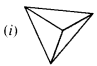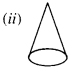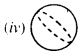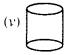NCERT Solutions for Class 6 Maths Chapter 5 Understanding Elementary Shapes Ex 5.9 are part of NCERT Solutions for Class 6 Maths. Here we have given NCERT Solutions for Class 6 Maths Chapter 5 Understanding Elementary Shapes Ex 5.9.

 Board CBSE Textbook NCERT Class Class 6 Subject Maths Chapter Chapter 5 Chapter Name Understanding Elementary Shapes Exercise Ex 5.9 Number of Questions Solved 2 Category NCERT Solutions

## NCERT Solutions for Class 6 Maths Chapter 5 Understanding Elementary Shapes Ex 5.9

### Chapter 5 Understanding Elementary Shapes Ex 5.9

Question 1.
Match the following :
(a) Cone(b) Sphere(c)
Cylinder(d)
Cuboid(e)
PyramidGive two new examples of each shape.
Solution :
(a) ↔ (ii)
(b) ↔ (iv)
(c) ↔ (v)
(d) ↔ (iii)
(e) ↔ (i).
(i) Birthday cap, ice-cream cone
(iv) Brick, book
(v) Pyramids of Egypt, right pyramid.

Question 2.
What shape is